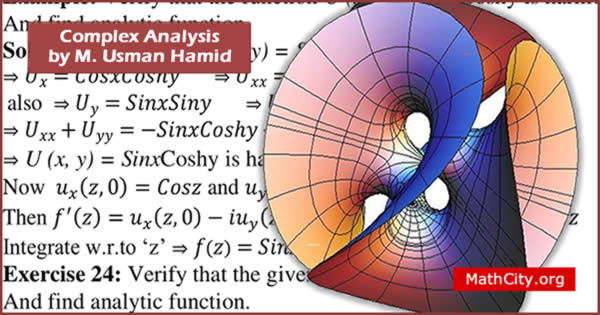# Complex Analysis by M Usman HamidWe are really very thankful to Muhammad Usman Hamid for providing these notes and appreciates his effort to publish these notes on MathCity.org

It covers the one part of the syllabus of Complex Analysis paper of MSc Mathematics. See the contents of the notes given below to see the topics covered by these notes.

The extension to the concept of complex numbers from that of real numbers was first necessitated by the solution of algebraic equations. For example, the quadratic equations $$x^2+4=0, x^2+x+1=0 \text{ and } x^2-2x+3=0$$ don't possess real roots.

In order to find the solution of these equations, Euler(1707-1783) was first to introduce the symbol $i=\sqrt{-1}$, this implies $i^2=-1$.

 Name Complex Analysis Muhammad Usman Hamid 182 pages PDF (see Software section for PDF Reader) 3.77 MB

The followings are the main topic in the notes. Please see or download PDF for details on topics.

• Order pair
• Complex numbers
• Vectors interpretation on complex numbers (graphical representation)
• The modulus or absolute value of complex number
• Complex conjugates
• Argument (amplitude) of complex numbers
• Exponential form
• Product and power in exponential form
• De Moivre's formula
• Arguments of product and quotients
• Roots of complex numbers
• How to find nth roots of complex numbers
• Regions in the complex plane
• Functions of a complex variable
• Single valued functons
• Multivalued functions
• Transformation
• Limits involving the point at infinity
• Continuity
• Uniform continuity
• Derivatives
• Analytics/Regular/Holomorphic functions
• Cauchy-Riemann equations in rectangular coordinates

Please click on View Online to see inside the PDF.

• notes/complex-analysis-m-usman-hamid